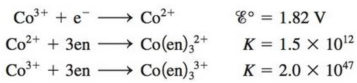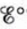# Consider the following data: where en = ethylenediamine. a. Calculate for the half-reaction b. Based on your answer to part a, which is the stronger oxidizing agent, Co 3+ or Co(en) 3 3+ ? c. Use the crystal field model to rationalize the result in part b.### Chemistry: An Atoms First Approach

2nd Edition
Steven S. Zumdahl + 1 other
Publisher: Cengage Learning
ISBN: 9781305079243

#### Solutions

Chapter
Section### Chemistry: An Atoms First Approach

2nd Edition
Steven S. Zumdahl + 1 other
Publisher: Cengage Learning
ISBN: 9781305079243
Chapter 20, Problem 91CP
Textbook Problem
3 views

## Consider the following data:where en = ethylenediamine.a. Calculatefor the half-reactionb. Based on your answer to part a, which is the stronger oxidizing agent, Co3+ or Co(en)33+?c. Use the crystal field model to rationalize the result in part b.

(a)

Interpretation Introduction

Interpretation: The standard electrode potential (Eο) for the reaction and stronger oxidizing agent among Co+3 and Co(en)33+ is to be stated. An explanation on basis of the crystal field model corresponding to the fact that one of the given two ions is the stronger oxidizing agent is to be stated.

Concept introduction: The electrons in the d orbital of a transition metal split into high and low energy orbitals when ligands are attached to it. The energy difference between these two levels depends upon the properties of both metal and the ligands. If the ligand is strong, then splitting will be high and the complex will be low spin. If the ligand is weak, then splitting will be less and the complex will be high spin.

To determine: The standard electrode potential (Eο) for the given half reaction.

### Explanation of Solution

Explanation

The given equations are,

Co+3+eCo+2Eο=1.82V (1)

Co2++3enCo(en)32+K=1.5×1012 (2)

Co3++3enCo(en)33+K2=2.0×1047 (3)

Where,

• Eο is the standard electrode potential for equation (1).
• K is the equilibrium constant of equation (2).
• K2 is the equilibrium constant of equation (3).

The equilibrium constant (K1) for equation (1) is calculated by the formula,

logK1=nEο0.0591

Where,

• K1 is the equilibrium constant of equation (1).
• n is the number of electrons.

The value of n is 1 .

Substitute the value of n and Eο in the above formula.

logK1=1×1.820.0591logK1=30.79K1=6.165×1030

Now the equation (1) becomes,

Co+3+eCo+2K1=6

(b)

Interpretation Introduction

Interpretation: The standard electrode potential (Eο) for the reaction and stronger oxidizing agent among Co+3 and Co(en)33+ is to be stated. An explanation on basis of the crystal field model corresponding to the fact that one of the given two ions is the stronger oxidizing agent is to be stated.

Concept introduction: The electrons in the d orbital of a transition metal split into high and low energy orbitals when ligands are attached to it. The energy difference between these two levels depends upon the properties of both metal and the ligands. If the ligand is strong, then splitting will be high and the complex will be low spin. If the ligand is weak, then splitting will be less and the complex will be high spin.

(c)

Interpretation Introduction

Interpretation: The standard electrode potential (Eο) for the reaction and stronger oxidizing agent among Co+3 and Co(en)33+ is to be stated. An explanation on basis of the crystal field model corresponding to the fact that one of the given two ions is the stronger oxidizing agent is to be stated.

Concept introduction: The electrons in the d orbital of a transition metal split into high and low energy orbitals when ligands are attached to it. The energy difference between these two levels depends upon the properties of both metal and the ligands. If the ligand is strong, then splitting will be high and the complex will be low spin. If the ligand is weak, then splitting will be less and the complex will be high spin.

To determine: The use to crystal field model to rationalise the result in part b.

### Still sussing out bartleby?

Check out a sample textbook solution.

See a sample solution

#### The Solution to Your Study Problems

Bartleby provides explanations to thousands of textbook problems written by our experts, many with advanced degrees!

Get Started

Find more solutions based on key concepts
What are the moral implications of using alcohol during pregnancy?

Understanding Nutrition (MindTap Course List)

Where would you expect storms to develop?

Fundamentals of Physical Geography

White blood cells include all except the following: phagocytes killer T-cells B-cells antibodies

Nutrition: Concepts and Controversies - Standalone book (MindTap Course List)

Complete each of the following reactions: a. b.

Chemistry for Today: General, Organic, and Biochemistry

What is the structure and function of the extracellular matrix?

Biology: The Dynamic Science (MindTap Course List)

What do the letters in the name MTBE stand for?

Organic And Biological Chemistry

Where would you look for water in our solar system?

Oceanography: An Invitation To Marine Science, Loose-leaf Versin

The gravitational force exerted by the Sun on the Earth holds the Earth in an orbit around the Sun. Let us assu...

Physics for Scientists and Engineers, Technology Update (No access codes included)

Convert 5.25 x 105 milligrams to kilograms.

Introductory Chemistry: An Active Learning Approach